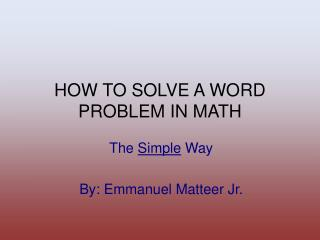# HOW TO SOLVE A WORD PROBLEM IN MATH - PowerPoint PPT PresentationDownload PresentationHOW TO SOLVE A WORD PROBLEM IN MATH

Download Presentation## HOW TO SOLVE A WORD PROBLEM IN MATH

- - - - - - - - - - - - - - - - - - - - - - - - - - - E N D - - - - - - - - - - - - - - - - - - - - - - - - - - -
##### Presentation Transcript

1. HOW TO SOLVE A WORD PROBLEM IN MATH The Simple Way By: Emmanuel Matteer Jr.

2. STEP #1—Read The Problem Carefully • Glance through to get an idea of what the problem is about. • Prepare to find and represent any known and unknown values.

3. STEP #2—DRAW A REPRESENTATION • Create either a chart or a diagram to enter your un/known values • Determine the type of illustration that is appropriate for use to the problem. [e.g., uniform motion, position, mixture, job, etc.,] • Complete the chart in full.

4. STEP #3—CREATE AND SOLVE THE EQUATION • Use the information from the chart to create the equation. • Then solve for the unknown value • IMPORTANT: Use common sense and logic when solving; for instance, don’t use any negative answers for problems that deal with real life [area, time, travel, distance].

5. STEP #4—ANSWER THE QUESTION • NOTE: Just because you solved the equation for the unknown doesn’t mean that it’s the correct answer! • Use the value that you have solved for to answer the question that they’re looking for. • Check your answer to see if it is correct.

6. SOME HELPFUL TIPS • Sometimes, take heed of how the teacher wants the problem solved. • Always include the units with your answers. • Be familiar with the rules of algebra, quadratic formula and other simple formulas, etc.